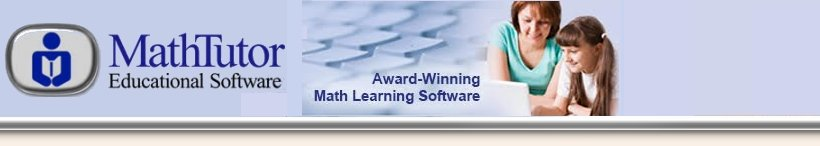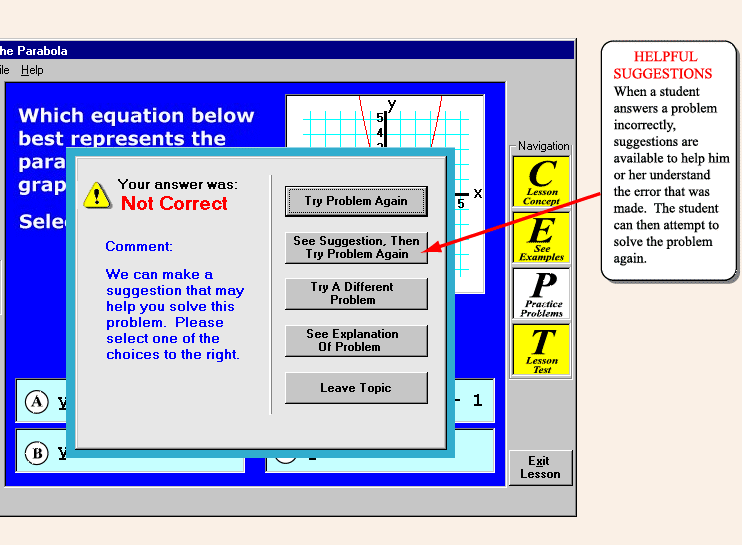# Sample Screen - Response to a Wrong Answer By Student

If a student's answer is incorrect, various options are available. The student can, for example, ask for a suggestion before trying the problem again. In some cases the program may suggest a particular approach to the problem. In other cases the program will attempt to analyze the student's answer, identify the error the student made, and point it out to the student.

Another option for students is to see an explanation of the problem. In this case, a complete step-by-step explanation is provided. The explanation will demonstrate all the steps involved in successfully solving problems, including: determining what information is given and what is being solved for; choosing variables to represent the quantities in the problem; setting up one or more equations based on the given information; solving the equation(s); and checking the results with the statement of the problem.×